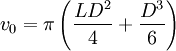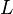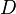# Difference between revisions of "Hard spherocylinders"

The hard spherocylinder model consists of an impenetrable cylinder, capped at both ends by hemispheres whose diameters are the same as the diameter of the cylinder. The hard spherocylinder model has been studied extensively because of its propensity to form both nematic and smectic liquid crystalline phases . One of the first studies of hard spherocylinders was undertaken by Cotter and Martire  using scaled-particle theory, and one of the first simulations was in the classic work of Jacques Vieillard-Baron . In the limit of zero diameter the hard spherocylinder becomes a line segment, often known as the hard rod model.

## Volume

The molecular volume of the spherocylinder is given by$v_0 = \pi \left( \frac{LD^2}{4} + \frac{D^3}{6} \right)$

where$L$ is the length of the cylindrical part of the spherocylinder and$D$ is the diameter.

## Minimum distance

The minimum distance between two spherocylinders can be calculated using an algorithm published by Vega and Lago . The source code can be found here. Such an algorithm is essential in, for example, a Monte Carlo simulation, in order to check for overlaps between two sites.

## Virial coefficients

Main article: Hard spherocylinders: virial coefficients

## Phase diagram

Main aritcle: Phase diagram of the hard spherocylinder model